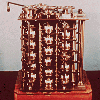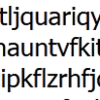#### You may also like### Route to Root

A sequence of numbers x1, x2, x3, ... starts with x1 = 2, and, if you know any term xn, you can find the next term xn+1 using the formula: xn+1 = (xn + 3/xn)/2 . Calculate the first six terms of this sequence. What do you notice? Calculate a few more terms and find the squares of the terms. Can you prove that the special property you notice about this sequence will apply to all the later terms of the sequence? Write down a formula to give an approximation to the cube root of a number and test it for the cube root of 3 and the cube root of 8. How many terms of the sequence do you have to take before you get the cube root of 8 correct to as many decimal places as your calculator will give? What happens when you try this method for fourth roots or fifth roots etc.?### Divided Differences

When in 1821 Charles Babbage invented the Difference Engine' it was intended to take over the work of making mathematical tables by the techniques described in this article.### Probably a Code?

Is the regularity shown in this encoded message noise or structure?

# Procedure Solver

### Why do this problem?

This problem is an introduction to algorithmic thinking. This branch of mathematical activity is of great use in decision mathematics and leads students into the important art of computer programming. It is essentially content free in terms of prior knowledge, so may be used at any stage of a course of study.

### Possible approach

This problem should be shown to the students with no explanation. What sense can they make of the layout and the problem? Gradually the concept that the problem represents an algorithm will emerge. Students may then begin to test the algorithm on pairs of numbers to try to determine what the procedure does. Can they prove that the algorithm does this in general?

Students might want to think algebraically. They might become confused by the assigments X = X - Y' and will need to understand that X represents a 'place holder' for a value which can change at each step of the procedure. Whilst such reassignements are common in computer programming, they might be seen as unusual by sixth formers.

### Key questions

This question demands clear organised thinking. Questioning should encourage this.
• What do the arrows and boxes represent?
• What happens, for example, for X = 10; Y = 25?
• What is the meaning of an expression such as 'X = X - Y'?

### Possible extension

• Can you program this procedure into Microsoft Excel?
• Can you create a clear, step-by-step algorithm to find the solution of a quadratic equation, taking into account that there might be 0, 1 or 2 distinct solutions?
• Pursue more formal ideas of logical programming using our LOGO environment .

### Possible support

If students are unused to the concept of an algorithm, you might first like to try the problems Flow Chart and Zeller's Birthday .